• python脚本，用于将matlab文件或matlab代码行转换为python。 该项目处于Alpha阶段。 此实现很大程度上取决于Victor Leikehman的项目。 当前的实现围绕着SMOP进行包装，但有以下区别： 它尝试生成不依赖libsmop代码，...
• 这些是“如何从 MATLAB 调用 Python”（ https://youtu.be/LHEZqpvE7D0 ）和“如何从 Python 调用 MATLAB”（ https://youtu.be/OocdPu1Tcrg ）视频的支持文件。 他们展示了通过结合 MATLABPython 功能对检测到...
• 支持面向对象和函数式编程 可扩展性，能调用C/C++代码 代码规范程度高，可读性强 目前几个比较流行的领域，Python都有用武之地。 云基础设施 - Python / Java / Go DevOps - Python / Shell / Ruby / Go 网络爬虫 - ...
• ## MATLAB 使用python代码

千次阅读 2018-12-24 11:21:05
matlab是一个非常强大的数学处理工具，python中很多工具库封装得非常好，对于高版本的MATLAB来说，可以直接使用python以及这些库，我这里使用的R2017a版本，网上说2015版开始就可以，没有测试，步骤如下： ...
matlab是一个非常强大的数学处理工具，python中很多工具库封装得非常好，对于高版本的MATLAB来说，可以直接使用python以及这些库，我这里使用的R2017a版本，网上说2015版开始就可以，没有测试，步骤如下：
1、将python添加至matlab里面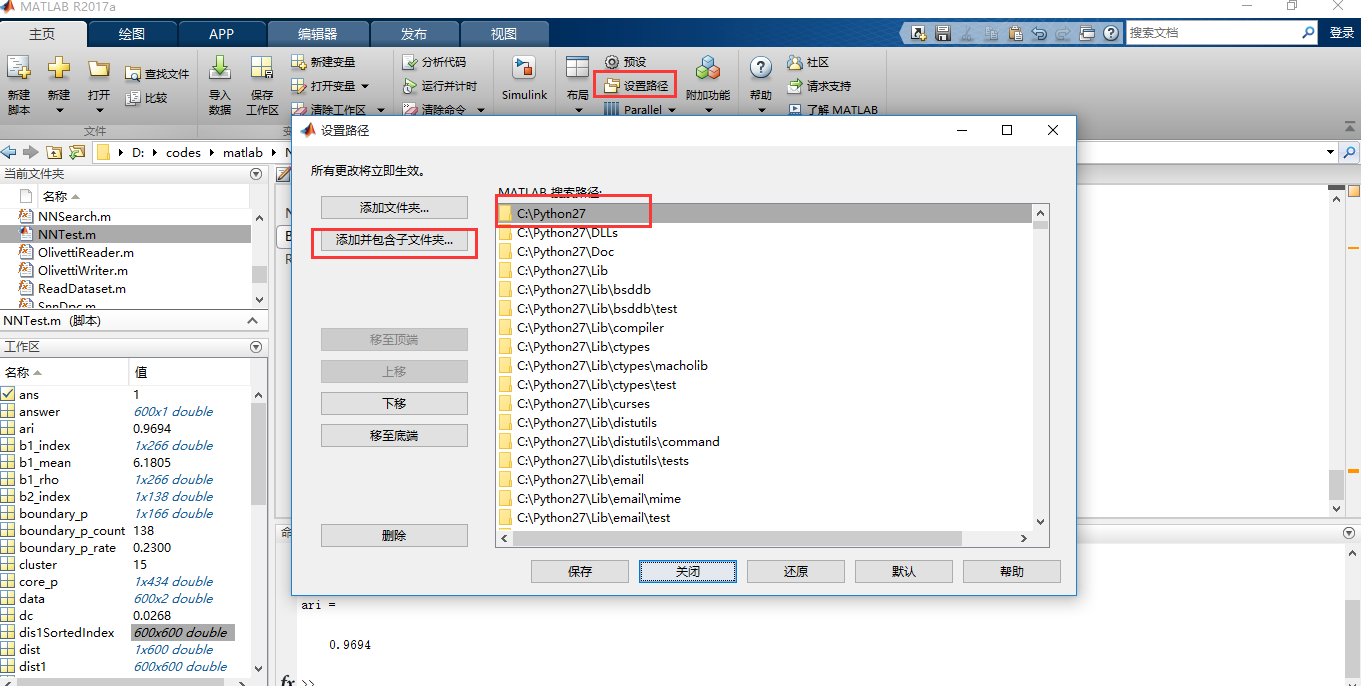2、使用python
使用时以 py.xxx来使用，比如使用list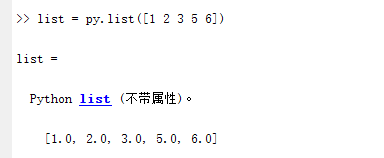使用库一样，比如我这里要使用sklearn计算NMI和ARI：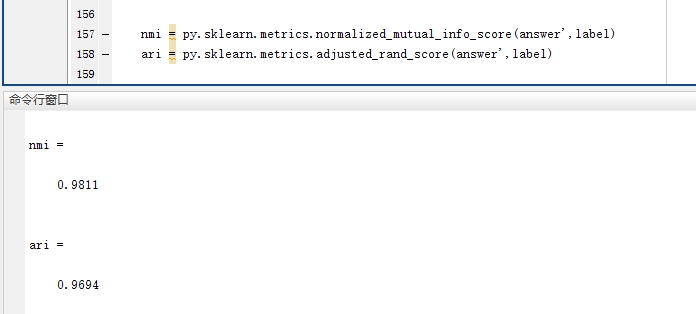展开全文• 支持面向对象和函数式编程 能够通过调用C/C++代码扩展功能 代码规范程度高，可读性强 目前几个比较流行的领域，Python都有用武之地。 云基础设施 - Python / Java / Go DevOps - Python / Shell / Ruby / Go 网络...
• win10下在jupyter notebook里添加matlab支持,解决python3.7不支持问题1.查看MATLAB Engine API for Python支持环境2.创建对应的虚拟环境1.更换conda为国内镜像源（自带的太慢）2.创建相应python版本的虚拟环境3.查看...


win10下在jupyter notebook里添加matlab支持,解决python3.7不支持问题
1.查看MATLAB Engine API for Python支持环境2.创建对应的虚拟环境1.更换conda为国内镜像源（自带的太慢）2.创建相应python版本的虚拟环境3.查看虚拟环境列表，激活，安装
3.安装matlab的setup.py1.安装setup.py2.安装ipykernel
4.安装nb_conda

1.查看MATLAB Engine API for Python支持环境

D:\MATLAB\R2016b\extern\engines\python

安装目录里找到python下的setup.py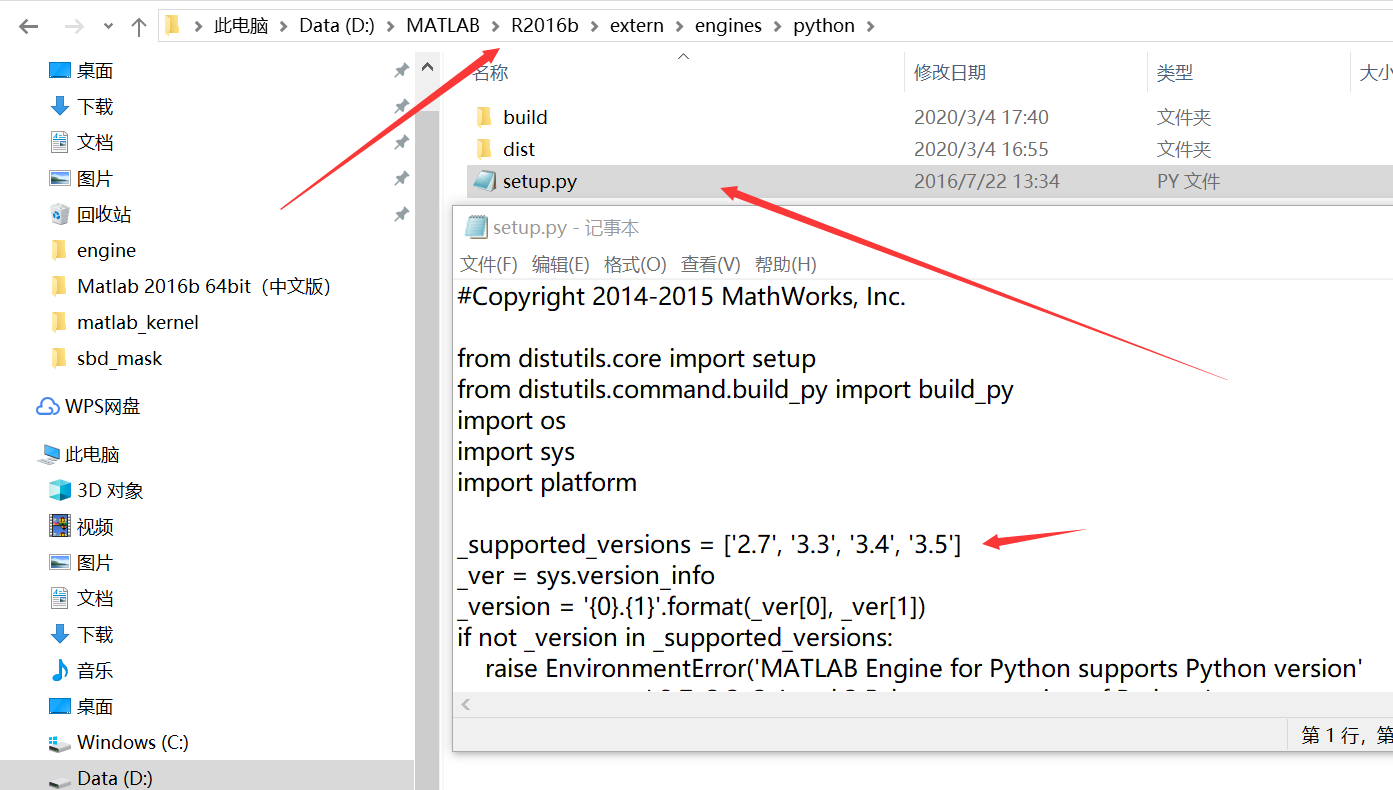我安装的是matlab2016b仅支持python3.5的环境 build是安装之后产生的目录，这里不用管
2.创建对应的虚拟环境
1.更换conda为国内镜像源（自带的太慢）
命令提示符里依次执行下面四条命令即可更换
conda config --add channels https://mirrors.tuna.tsinghua.edu.cn/anaconda/pkgs/free/
conda config --set show_channel_urls yes

2.创建相应python版本的虚拟环境
conda create -n python35 python=3.5

这里我创建了名为python35，python版本为3.5 python=X.X，可为3.6,3.7等相应的python环境
3.查看虚拟环境列表，激活，安装
查看

conda env list

激活环境,activate后面跟你的环境名

activate pyhon35

安装matlab_kernel, -i及后面的是临时使用镜像源下载
pip install matlab_kernel -i https://pypi.tuna.tsinghua.edu.cn/simple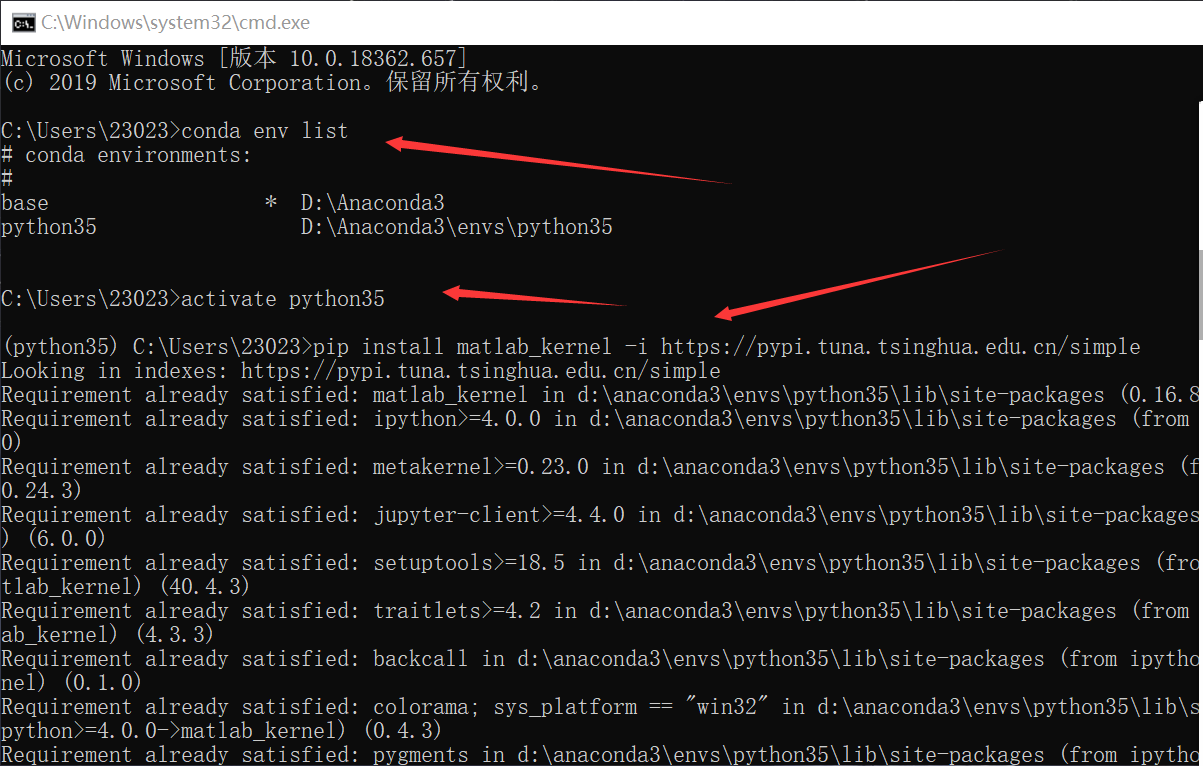3.安装matlab的setup.py
1.安装setup.py
切换到支持的虚拟环境之后 d:可切换到D盘

d:

cd 到setup.py的目录

cd D:\MATLAB\R2016b\extern\engines\python

然后执行

python3 setup.py install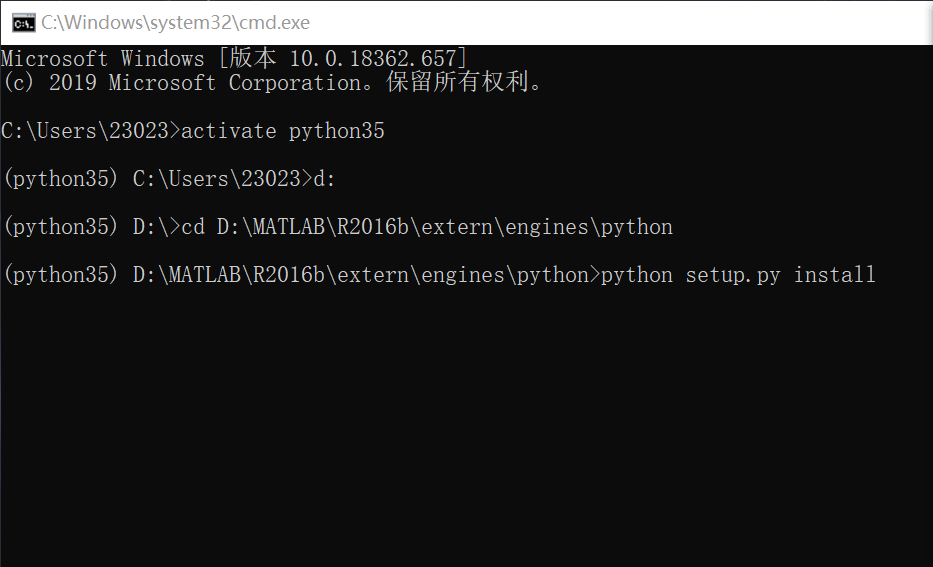这样就安装好了matlab
2.安装ipykernel
1.安装

pip install ipykernel -i https://pypi.tuna.tsinghua.edu.cn/simple

2.查看内核列表

jupyter kernelspec list

3.删除

jupyter kernelspec remove {name}

如下我们就能看到有matlab的内核，但这只是在虚拟环境里 虚拟环境里运行jupyter notebook会自动跳到base环境的路径 这样打开jupyter还是没有matlab，这就需要下面的插件解决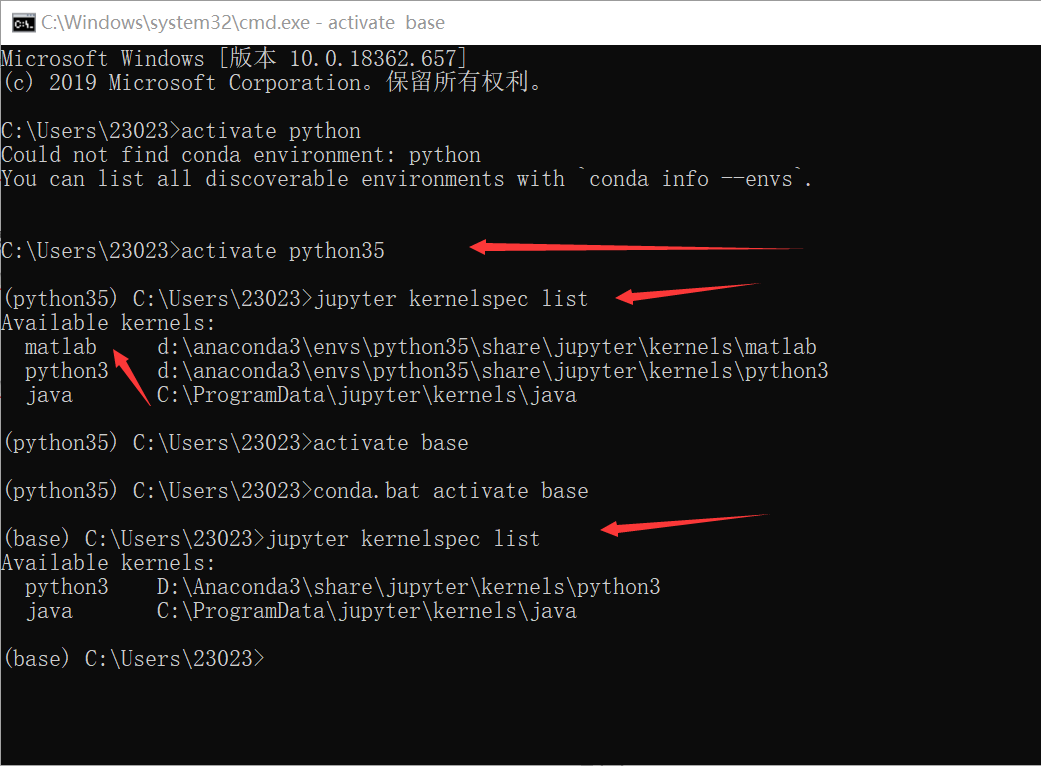4.安装nb_conda
切换到主环境（base）之后安装

conda install nb_conda

再次打开jupyter之后，就可以找到了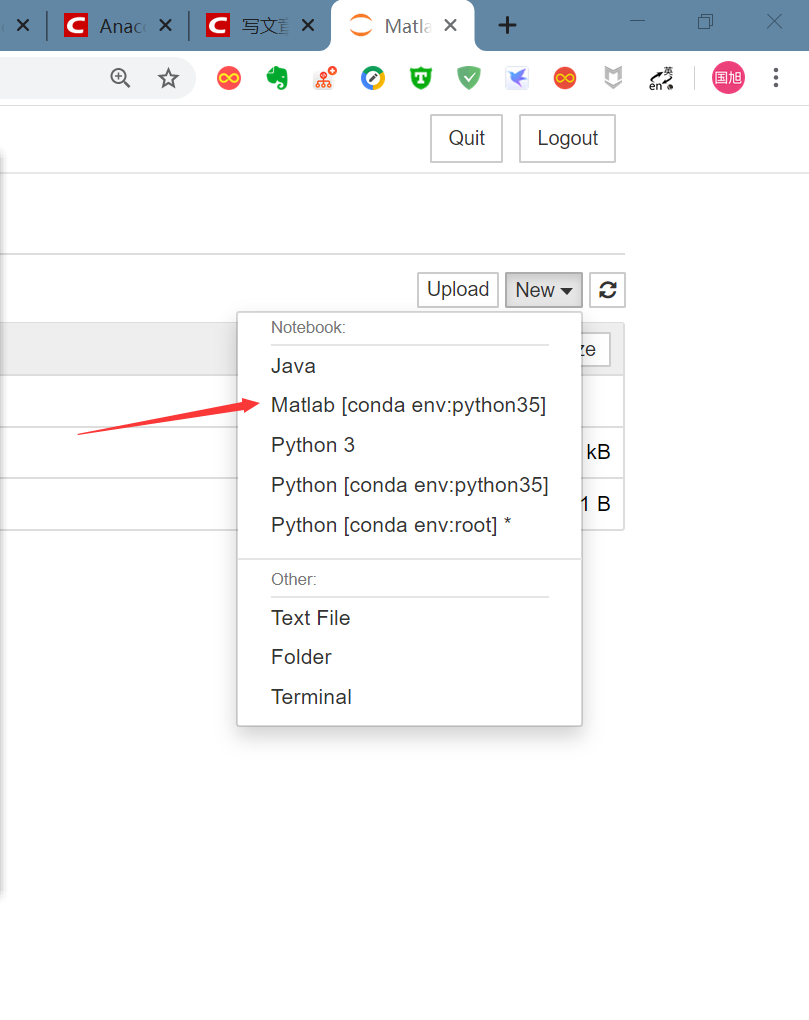大功告成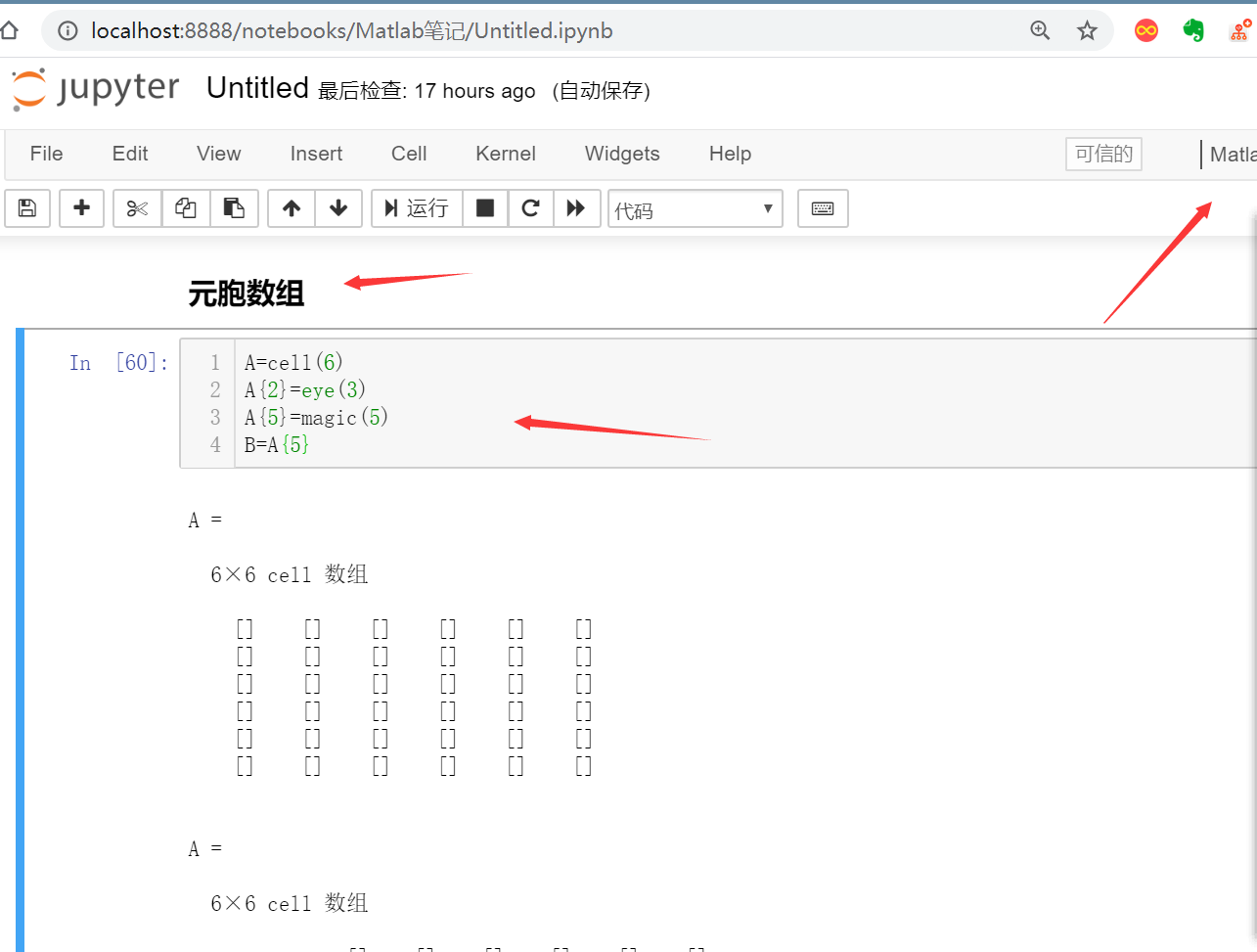有关于 win10下安装Anaconda，并配置jupyter notebook和修改默认打开路径 ，参考 这儿.
展开全文anaconda 机器学习
• 注意的是python和matlab版本有对应关系，我下载了matlab2010和2014，前者没有engines文件夹，后者只支持python2.7和3.3！ 已知matlab2017b支持python3.6 ~下载对应破解版，安装matlab后再参考调用的文...
调用的话参考
https://blog.csdn.net/m0_37316917/article/details/90256563
https://zhuanlan.zhihu.com/p/67127872
注意的是python和matlab版本有对应关系，我下载了matlab2010和2014，前者没有engines文件夹，后者只支持python2.7和3.3！
已知matlab2017b支持python3.6    ~下载对应破解版，安装matlab后再参考调用的文章.

环境：Windows10，Matlab R2018，Pycharm
1. 安装 新建python工程，工程目录为 E:\untitled Matlab为Python提供了专门的Matlab引擎，在Pycharm中打开Terminal，定位到Matlab引擎所在的目录：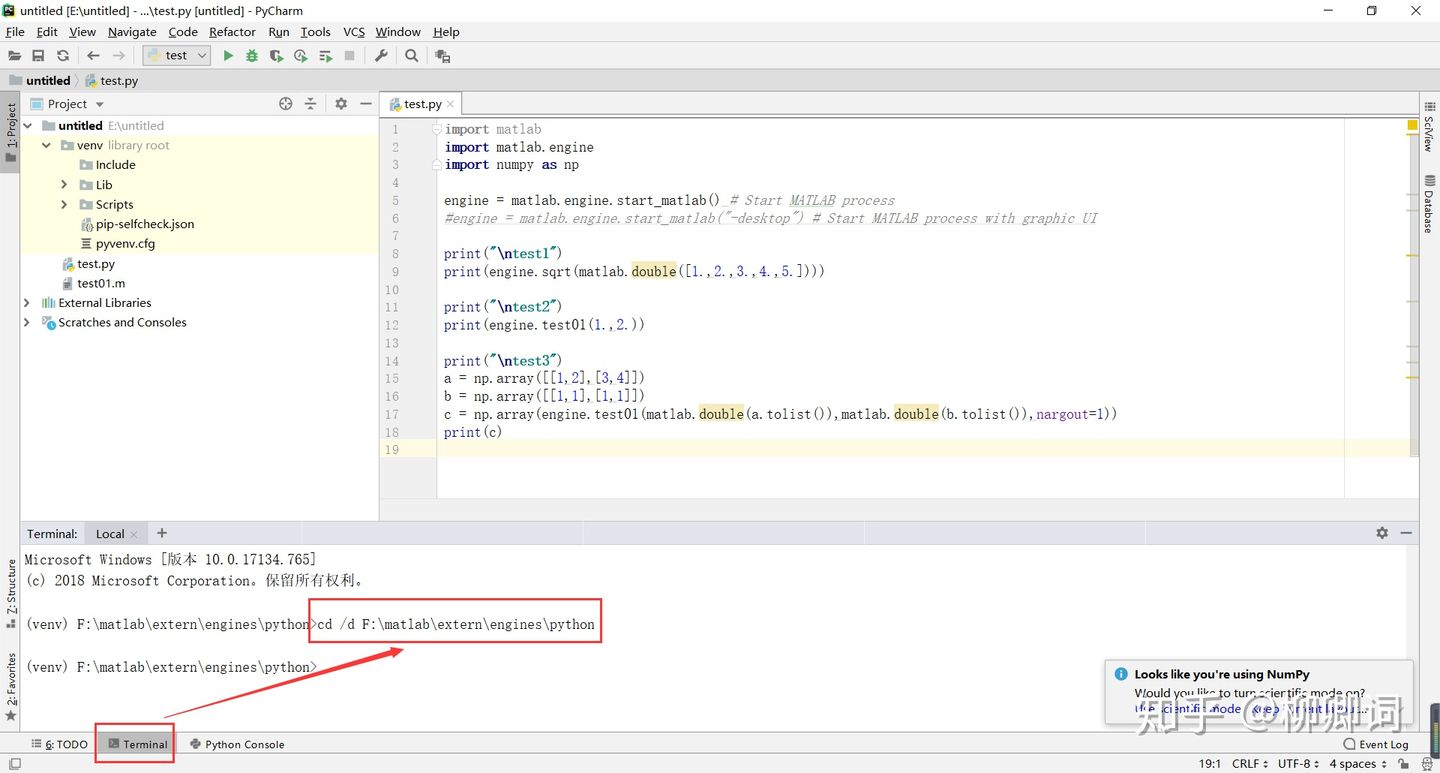Pycharm的Terminal位于界面的左下角，与Anaconda Prompt具有相同的功能，可以执行pip命令
本人的目录为 F:\matlab\extern\engines\python，其中extern前是matlab安装目录，后续是固定目录 输入python setup.py install安装Matlab引擎
2. 导入Matlab引擎与使用 输入如下命令导入matlab.engine ：
import matlab
import matlab.engine
matlab函数在python调用中，首先需要启动一个engine，通过engine去启动matlab函数，也可以启动多个引擎来，启动多个计算，也可以使用非同步启动等方式，这些在官网中都有说明： [https://ww2.mathworks.cn/help/matlab/matlab_external/start-the-matlab-engine-for-python.html]
engine = matlab.engine.start_matlab() #启动matlab
（1）调用matlab系统函数
 engine.sqrt(matlab.double([1.,2.,3.,4.,5.]))
（2）调用m文件
在工程目录（python源程序文件同目录）下新建matlab源程序文件，具体如下：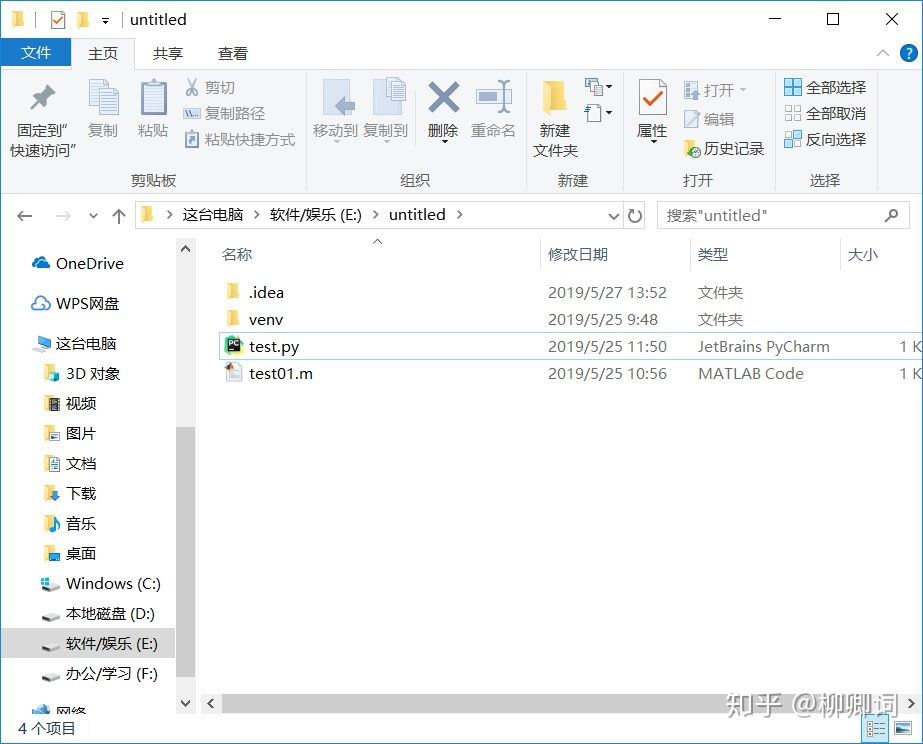matlab源程序文件与python源程序文件处于同一目录下即可
文件名为test01.m，写入如下代码：
function a=test01(a,b)
a=0.5*(a+b)
python中调用：
engine.test01(1.,2.)
注意事项
（1）数据类型差异 engine传入时，需要将python的数据类型转换成matlab可用。 以常用的numpy数组为例： a = np.array([[1,2],[3,4]]) ，传入时需要使用matlab.double(a.tolist)的形式， 同时engine的输出也是matlab对象，需要进行一定的处理。
测试代码如下：
a = np.array([[1,2],[3,4]])
b = np.array([[1,1],[1,1]])
c = np.array(engine.test01(matlab.double(a.tolist()),matlab.double(b.tolist()),nargout=1))
print(c)
（2）返回值个数
如上述代码中nargout=1，对应test01函数返回值个数，默认为1个，0个或多个返回值需要对应修改，否则会报错
（3）资源回收
 engine.exit()
运行结果附图：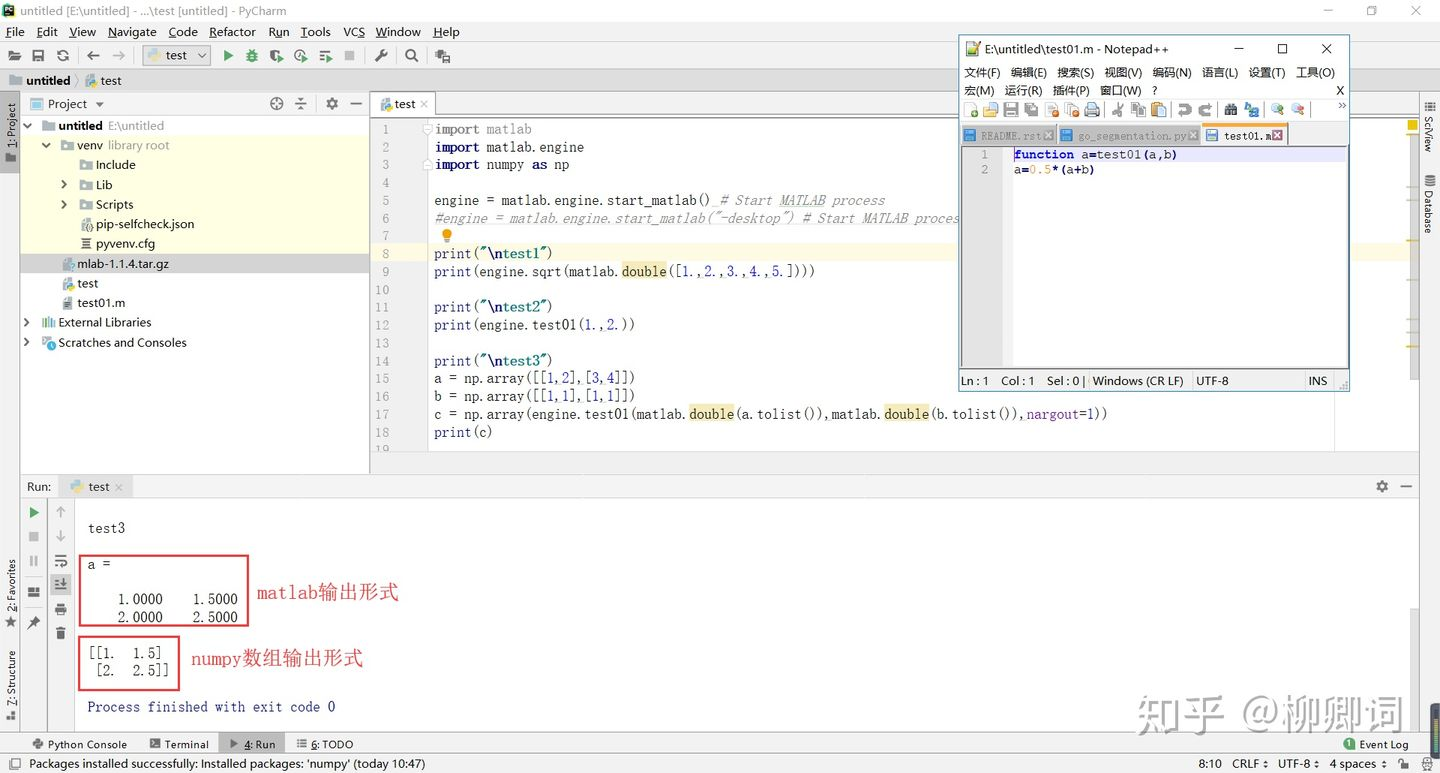结合调用m文件、输入数据转换、返回值个数控制与输出数据转换
展开全文• ## MATLAB调用python实现混合编程

千次阅读 多人点赞 2020-02-27 02:00:00
MATLAB调用python实现混合编程 MATLAB是学术界最常用的编程工具，虽然MATLAB的功能已经很强大了，但是相对于开源的python来说，python丰富的开源工具和框架也是MATLAB所望尘莫及的。在编程实现任务所需的功能时，...
                             MATLAB调用python实现混合编程

MATLAB是学术界最常用的编程工具，虽然MATLAB的功能已经很强大了，但是相对于开源的python来说，python丰富的开源工具和框架也是MATLAB所望尘莫及的。在编程实现任务所需的功能时，可能你对MATLAB相当熟悉，但是MATLAB里面却没有现成的代码供你使用，而恰好python却有开源的代码实现（比如当今正火的sklearn，比如pytorch等等等等），这时很头疼的情况就出现了----你对python不熟悉！！！
就问你难不难受？
别慌，你看----他来了，他带着混合编程走来了，他就是MATLAB与python混合编程！
下面，我们直奔主题————>怎么编程？

首先，你需要知道，MATLAB想要与python实现混合编程是有条件滴，条件就是MATLAB版本至少在2016版本以上（当然这只是As I all know，其实不太准确，因为我也是从网上看到的，反正2017版本是可以的）。
然后我们正式开始。
首先甩条官方链接：https://ww2.mathworks.cn/help/matlab/getting-started-with-python.html
作为一名程序猿，你得知道，新东西搞不懂？---->看文档
没错，我就是因为网上啥都找不到，最后实在无奈才去看的文档。（虽然官网文档能打开，但响应速度实在感人，所以下面我会直接将部分文档内容复制下来，省去你等待的时间，如果还是看不懂，你可以选择自己去官网看）
一、入门
想要实现MATLAB和python的混合编程，那MATLAB和python你必须得有，环境变量配置好，这时前提，然后在MATLAB的命令行窗口输入：
pyversion
然后会显示当前的python信息。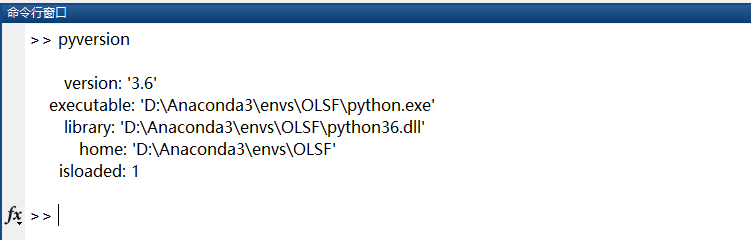如果没有显示，就手动指定。
使用下面命令，后面的是你电脑python.exe所在的绝对路径，如果你的电脑上有不止一个python环境（比如用anaconda创建了多个虚拟环境），也可以使用下面的命令来切换到不同的python环境
pyversion D:\Anaconda3\envs\OLSF\python.exe
不过执行之后什么输出都没有，如下：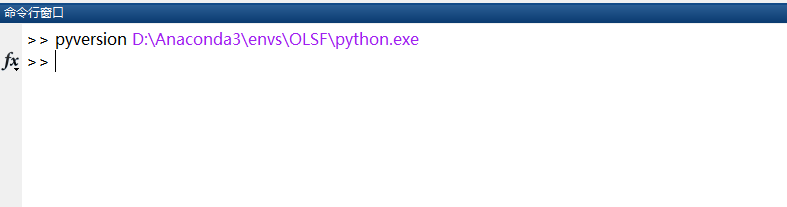有一点很必要知道的是，前面的命令（包括后面的命令，比如导包）执行完之后，MATLAB会将python的信息什么的加载到内存中去，我看网上说此时如果修改了代码什么的以后，重新执行，你会发现MATLAB调用的仍然是没修改之前的代码，修改没起到作用，此时你需要重启MATLAB，或者执行重新加载命令，看完文档之后我发现，还有一种方法可以不用重启MATLAB，那就是清除缓存！
下面先说怎么清除缓存，重新加载后面会说到。
#清楚缓存，包括工作区变量和已经加载的类和python模块缓存
clear classes

下面的内容来自官方文档：
------------------------------------------------------------------------我是一条分割线------------------------------------------------------------------------
快速入门
有助于您快速开始在 MATLAB® 中使用 Python® 的示例和概念
要从 MATLAB 中调用 Python 库，请安装受支持的 Python 参考实现 (CPython) 版本。MATLAB 支持版本 2.7、3.5、3.6 和 3.7。您需要安装的版本取决于需要使用的库。有关信息，请参阅安装支持的 Python 实现。
要调用 Python 函数，请在模块名称和函数名称前键入 py.。将 MATLAB 数据作为参数传递给 Python 函数；MATLAB 会将数据转换为最适合在 Python 语言中表达该数据的类型。例如，py.os.listdir('.') 列出当前文件夹的内容。
如需关于 Python 语言的帮助，请参阅 www.python.org/doc。如需关于第三方模块或用户定义模块的帮助，请参考产品文档。
要从 Python 应用程序调用 MATLAB 函数，请参阅从 Python 调用 MATLAB。
函数
pyversion更改 Python 解释器的默认版本
类
matlab.exception.PyException捕获 Python 异常的错误信息
主题
系统和配置要求
如何验证您已安装受支持的 Python 版本。
创建一个 Python 对象。
MATLAB 变量和 Python 对象的行为有所不同。
Help for Python Functions
How to find help for Python functions.
了解 Python 和 MATLAB import 命令
如何使用 Python import 语句和 MATLAB import 命令。
调用用户定义的 Python 模块
创建本文档中的示例使用的一个 Python 模块。
重新加载经过修改的用户定义的 Python 模块
此示例说明如何重新加载经过修改的 Python 模块。
安装支持的 Python 实现
如何从 www.python.org 网站安装支持的 Python 版本。
------------------------------------------------------------------------我也是一条分割线------------------------------------------------------------------------
二、创建python对象
这部分直接看官方文档就行，说白了创建对象就等于python中 的实例化一个类，不过如果你想修改类的属性默认值的话，只能用创建的对象点儿（.）属性名=value的方式来修改。
------------------------------------------------------------------------我还是一条分割线------------------------------------------------------------------------
创建一个 Python 对象。
创建 Python® 对象 pyObj 的语法如下：
pyObj = py.modulename.ClassName(varargin)
其中 varargin 是由 ClassName 中的 __init__ 方法指定的构造函数参数列表。
在 MATLAB® 中，Python 对象是引用类型（句柄对象），并且不遵从 MATLAB 的“赋值时复制”和“传值”规则。当您复制句柄对象时，只会复制句柄并且旧句柄和新句柄都引用相同的数据。当您复制 MATLAB 变量（值对象）时，变量数据也会复制。更改原始变量并不会影响新变量。
以下示例在 Python 标准库 textwrap 模块中创建 TextWrapper 类的一个对象。
读取构造函数签名 __init__。
py.help('textwrap.TextWrapper.__init__')
Help on method __init__ in textwrap.TextWrapper:

textwrap.TextWrapper.__init__ = __init__(self, width=70, initial_indent='', subsequent_indent='', expand_tabs=True, replace_whitespace=True, fix_sentence_endings=False, break_long_words=True, drop_whitespace=True, break_on_hyphens=True) unbound textwrap.TextWrapper method

创建一个默认 TextWrapper 对象。您不需要传递任何输入参数，因为每个参数都有由等号 (=) 字符标识的默认值。
tw = py.textwrap.TextWrapper;
tw =

Python TextWrapper with properties:

width: 70
subsequent_indent: [1x1 py.str]
wordsep_simple_re_uni: [1x1 py._sre.SRE_Pattern]
fix_sentence_endings: 0
break_on_hyphens: 1
break_long_words: 1
wordsep_re_uni: [1x1 py._sre.SRE_Pattern]
initial_indent: [1x1 py.str]
expand_tabs: 1
replace_whitespace: 1
drop_whitespace: 1

<textwrap.TextWrapper instance at 0x000000006D58F808>
要更改某个逻辑值，例如 break_long_words 属性，请键入：
tw.break_long_words = 0;
要更改某个数值，例如 width 属性，请先确定数值类型。
class(tw.width)
ans =

int64
默认情况下，当您将 MATLAB 数字传递给 Python 函数时，Python 将其作为浮点数读取。如果函数需要的是整数，Python 可能会引发错误或产生意外的结果。将 MATLAB 数字显式转换为整数。例如，键入：
tw.width = int64(3);
阅读 wrap 方法的帮助。
py.help('textwrap.TextWrapper.wrap')
Help on method wrap in textwrap.TextWrapper:

textwrap.TextWrapper.wrap = wrap(self, text) unbound textwrap.TextWrapper method
wrap(text : string) -> [string]

Reformat the single paragraph in 'text' so it fits in lines of
no more than 'self.width' columns, and return a list of wrapped
lines.  Tabs in 'text' are expanded with string.expandtabs(),
and all other whitespace characters (including newline) are
converted to space.
从输入 T 创建换行的列表 w。
T = 'MATLAB® is a high-level language and interactive environment for numerical computation, visualization, and programming.';
w = wrap(tw,T);
whos w
  Name      Size            Bytes  Class      Attributes

w         1x1               112  py.list   
将 py.list 转换为元胞数组并显示结果。
wrapped = cellfun(@char, cell(w), 'UniformOutput', false);
fprintf('%s\n', wrapped{:})
MATLAB®
is
a
high-
level
language
and
interactive
environment
for
numerical
computation,
visualization,
and
programming.
尽管 width 为 3，但将 break_long_words 属性设置为 false 会覆盖显示中的 width 值。
------------------------------------------------------------------------我又是一条分割线------------------------------------------------------------------------
三、导包（import命令）和调用模块
MATLAB想要导入python的包很简单，python里导包是这么导：
import numpy as np
from sklearn.metrics import f1_score
而用MATLAB调用python包时只需要在前面加个py.就行，比如：
import py.numpy
import py.torch.*
如果要导入自己写的模块，就这么写：
# mymodule是你写的模块的文件名比如：mymodule.py
# myfunc是mymodlue.py里面的函数
py.mymodule.myfunc(参数1，参数2，...)
这里要注意的是：你自己写的模块必须放在python的搜索路径下，让python可以找到他，或者直接放在你MATLAB代码的目录下。当放在MATLAB代码目录下时，需要将当前文件夹添加到 Python 搜索路径，执行如下命令：
if count(py.sys.path,'') == 0
insert(py.sys.path,int32(0),'');
end
然后看官方文档：
------------------------------------------------------------------------我又是一条分割线------------------------------------------------------------------------
了解 Python 和 MATLAB import 命令
import 语句在 MATLAB® 中的功能与在 Python® 中的功能不同。Python 使用 import 语句加载代码并使代码可供访问。MATLAB 使用 import 函数来引用类或函数，而不必使用包名称。
小心
切勿调用：
import py.*
如果执行了此调用，则 MATLAB 会调用 Python 函数，而不是同名的 MATLAB 函数。这可能会导致意外的行为。
如果您调用此命令，则必须调用 MATLAB 命令：
clear import
请勿键入 import pythonmodule
当您键入以下内容时，MATLAB 会自动加载 Python：
py.command
请勿在 MATLAB 中键入：
import pythonmodule
使用 MATLAB import 缩短类或函数名称
借助 Python from...import 语句，您可以在不使用完全限定名称的情况下引用模块。假设您有以下 Python 代码，其中 y 是您要使用的类或函数名称。
from x import y
将此语句替换为以下 MATLAB 代码：
import x.y
例如，Python textwrap 模块会格式化文本块。
S = py.textwrap.wrap('This is a string');
由于 wrap 不是 MATLAB 函数，您可以使用 import 函数缩短调用语法。调用此命令后，您不需要键入包 (py) 和模块 (textwrap) 名称。
import py.textwrap.wrap
S = wrap('This is a string');
创建 Python 模块
将当前文件夹更改为可写文件夹。在 MATLAB 编辑器中打开一个新文件。
复制下列用于定义 myfunc 函数的语句并将文件另存为 mymod.py。
def myfunc():
"""Display message."""
return 'version 1'

调用 myfunc。
py.mymod.myfunc
ans =

Python str with no properties.

version 1
修改模块
修改该函数，用以下代码替换 return 语句：
    return 'version 2'
保存文件。
卸载模块
clear classes

MATLAB 删除工作区中的所有变量、脚本和类。
导入经过修改的模块
mod = py.importlib.import_module('mymod');

在 Python 版本 2.7 中重新加载模块
py.reload(mod);

在 Python 版本 3.x 中重新加载模块
py.importlib.reload(mod);

调用更新后的模块中的函数
调用更新后的 myfunc 函数。
py.mymod.myfunc
ans =

Python str with no properties.

version 2
调用用户定义的 Python 模块
此示例说明如何从以下 Python® 模块调用方法。本文档中的示例会使用此模块。
此示例说明如何在 MATLAB® 中创建该模块。如果您在 Python 编辑器中创建 mymod.py，请确保该模块位于 Python 搜索路径上。此示例还可指导经验不丰富的 Python 用户如何获得调用该函数的帮助。
将当前文件夹更改为可写文件夹。
在 MATLAB 编辑器中打开一个新文件。
复制下列命令并将该文件另存为 mymod.py。
# mymod.py
"""Python module demonstrates passing MATLAB types to Python functions"""
def search(words):
"""Return list of words containing 'son'"""
newlist = [w for w in words if 'son' in w]
return newlist

def theend(words):
"""Append 'The End' to list of words"""
words.append('The End')
return words

从 MATLAB 命令提示符下，将当前文件夹添加到 Python 搜索路径。
if count(py.sys.path,'') == 0
insert(py.sys.path,int32(0),'');
end
要了解如何调用函数，请阅读 mymod.py 源文件中 search 函数的函数签名。该函数接受一个输入参数 words。
def search(words):
阅读 mymod.py 源文件中的函数帮助。根据 Python 网站文档，帮助位于“作为模块、函数、类或方法定义中的第一条语句出现的字符串文字”中。search 的帮助是：
"""Return list of words containing 'son'"""
该函数返回一个列表。
在 MATLAB 中创建一个输入参数，即名称列表。
N = py.list({'Jones','Johnson','James'})
N =

Python list with no properties.

['Jones', 'Johnson', 'James']
调用 search 函数。在模块名称和函数名称前键入 py.。
names = py.mymod.search(N)
names =

Python list with no properties.

['Johnson']
该函数返回 py.list 值。
原始输入 N 未更改。
N
N =

Python list with no properties.

['Jones', 'Johnson', 'James']
------------------------------------------------------------------------我又是一条分割线------------------------------------------------------------------------
四、MATLAB与python数据类型的映射关系
------------------------------------------------------------------------我又是一条分割线------------------------------------------------------------------------
列表、元组和字典类型
下表显示用于创建 list、tuple 和 dict 类型的命令。左侧的命令从 Python® 解释器运行。右侧的命令是 MATLAB® 命令。
Python list - []  MATLAB py.list ['Robert', 'Mary', 'Joseph']py.list({'Robert','Mary','Joseph'})[[1,2],[3,4]]py.list({py.list([1,2]),py.list([3,4])})
Python tuple - ()  MATLAB py.tuple ('Robert', 19, 'Biology')py.tuple({'Robert',19,'Biology'})
Python dict - {}  MATLAB py.dict {'Robert': 357, 'Joe': 391, 'Mary': 229}py.dict(pyargs(... 'Robert',357,'Mary',229,'Joe',391))
------------------------------------------------------------------------我又是一条分割线------------------------------------------------------------------------
五、函数（这部分还没有深入研究）
先看下官方文档：
------------------------------------------------------------------------我又是一条分割线------------------------------------------------------------------------
要调用 Python 方法或函数，请键入 py.，然后键入模块名、函数名和参数。
在大多数情况下，MATLAB 自动将输入参数转换为 Python 类型。有一个例外情况是用关键字参数调用 Python 函数时。这种情况下需要使用 pyargs 函数将 MATLAB 数据传递给这些函数。
------------------------------------------------------------------------我又是一条分割线------------------------------------------------------------------------
这里有必要说一下，在python中一般使用的内置函数或者框架函数都会有多个可以省略的形参，比如这种形式：
def f1_score(y_true, y_pred, labels=None, pos_label=1, average='binary',
sample_weight=None):
像这种形式的函数在python中是极其常见的，而我们在调用的时候一般会省略其中一些参数，比如这么写：
result = f1_score(y_true, y_pred, average="macro")
这时中间的pos_label等参数会被省略，省略写的时候就需要将后面的参数明确 的标出来比如后面的average= ‘macro’，但是这种调用在MATLAB中使用时非法的，系统会报错。我在网上冲了很久很久的浪也没发现怎么解决这个问题，最后干脆自己想了个办法，自己写个python函数来实现相同的效果。以上面这个函数为例，我新建了一个module.py的文件，然后在里面重写了一下，上代码：
# module.py

from sklearn.metrics import f1_score

def F1_score(y_true, y_pre, average):
return f1_score(y_true, y_pre, average=average)
这样一来，我就可以通过py.module.F1_score()来调用sklearn的f1_score函数了。

六、捕获异常
在python中是存在异常的，但是当MATLAB调用python代码时，MATLAB并不会自动捕获python的异常，这就需要手动去捕获python代码运行产生的异常。
直接看官方文档：
------------------------------------------------------------------------我又是一条分割线------------------------------------------------------------------------
matlab.exception.PyException 类
包： matlab.exception
捕获 Python 异常的错误信息
全页展开
说明
处理 matlab.exception.PyException 对象中的信息，以解决从 MATLAB® 调用的 Python® 方法引发的 Python 错误。此类派生自 MException。
创建对象
您不要显式构造 matlab.exception.PyException 对象。在 Python 引发异常时，MATLAB 会自动构造一个 PyException 对象。PyException 对象将包装原始的 Python 异常。
属性
全部折叠
ExceptionObject - 对象 异常对象
Python sys.exc_info 函数的结果。要了解函数返回的内容信息，请键入：
help('py.sys.exc_info')

exc_info() -> (type, value, traceback)

Return information about the most recent exception caught by an except
clause in the current stack frame or in an older stack frame.

示例
全部折叠
捕获 Python 异常
生成一个 Python 异常并显示信息。当 MATLAB 显示包含文本 Python Error 的消息时，请参阅您的 Python 文档以了解详细信息。
try
py.list('x','y',1)
catch e
e.message
if(isa(e,'matlab.exception.PyException'))
e.ExceptionObject
end
end
ans =

Python Error: TypeError: list() takes at most 1 argument (3 given)

ans =

Python tuple with no properties.

(<type 'exceptions.TypeError'>, TypeError('list() takes at most 1 argument (3 given)',), None)
------------------------------------------------------------------------我又是一条分割线------------------------------------------------------------------------
文档写的已经很清楚了，MATLAB也是通过类似python的try-catch机制进行异常的捕获，只不过想要将异常信息抛出，需要添加一个if判断，来判断该异常是否是python的异常。

到这里MATLAB与python的混合编程就结束了，总结一下就是不懂得多看文档，文档说的比别人说的更准确更简洁。
还有就是，多动手，编程不是想想就行，好记性不如烂键盘！

欢迎讨论，欢迎转载！！！
转载请注明出处，谢谢！

欢迎访问我的个人博客：机器学习之路
展开全文机器学习
• 接口支持两个函数fastwht和had_mat_idx 。 fastwht函数是 MATLAB 的fwht函数的更快版本，对于它的用法，我们参考 MATLAB 自带的 . 这是一个在 2^nx 2^n 序列有序哈达玛矩阵中生成请求条目的函数。 当您想在单个或几...
• python调用m文件需要安装matlab.engine，注意matlab版本与python版本的兼容性，一般matlab支持python3.6以下版本，matlab2020b支持python3.8，可以通过命令窗口查看python版本，开始-->运行-->输入“cmd”，...
• 使用方法2.1 数据映射问题MATLABPython传递数据MATLAB 类型到 Python 类型的映射MATLAB 向量到 Python 的映射传递矩阵和多维数组处理从 Python 返回的数据Python 类型到 MATLAB 类型的自动映射显式类型转换2.2 ...
• 支持面向对象和函数式编程，动态语言 能够通过调用C/C++代码扩展功能 代码规范程度高，可读性强 目前几个比较流行的领域，Python都有用武之地。 云基础设施 - Python / Java / Go DevOps - Python / Shell / Ruby / ...
• pyplotz-0.24 pyplotz-0.24 pythonmatlab绘图支持中文的插件，使用方式请移步博文：https://blog.csdn.net/xiaocy66/article/details/83110220
• 典型相关分析matlab实现代码 python ======== python资源集合，持续更新。。。 。 目录 ======== 具体内容 ============================= ======== 网页框架 Django - Django。 - Channels旨在增强Django的异步能力...
• matlab R2016b 注意：matlab2016 支持python最高版本为3.5 步骤1: 进入此文件夹 运行命令： python setup.py install 步骤二： pip 安装各种包 步骤三： 你就可以使用呢 接下里验张pythonMATLAB 混编...
• 很多时候，我们需要把matlab里的数据保存下来，然后用python来处理。方法一(.mat格式)直接将matlab的数据存储成.mat格式，然后在python中利用scipy.io中的loadmat函数来读取import scipy.io as sio data = sio....数据 数据存储
• LRP工具箱为支持Matlabpython的人工神经网络提供了简单且可访问的LRP独立实现。 工具箱实现了Caffe深度学习框架的LRP功能，作为10/2015年发布的Caffe源代码的扩展。 Matlabpython的实现旨在用作沙箱或游乐场，以...
• 支持面向对象和函数式编程 可扩展性，能调用C/C++代码 代码规范程度高，可读性强 目前几个比较流行的领域，Python都有用武之地。 云基础设施 - Python / Java / Go DevOps - Python / Shell / Ruby / Go 网络爬虫 - ...
• 因为matlab支持 32bitpython 和高版本python。而系统默认装是32bit 。anaconda只能虚拟路径。 一：添加anaconda的envs到path 代替系统的32bit 二：装个64bit的python 三：在matlab中指定路径。重启后会变 ...anaconda
• python 下与matlab下SVM 使用结果对比： 1、matlab测试code: scores_train = ones(28,1)*0.8 scores_train(1,1)=6 scores_train(2,1)=4 score_size= size(scores_train) D=ones(28,5)*0.2 scores_...SVR SVM
• MatlabPython 之间的转换 有几个替代工具用于将Matlab代码转换为Python代码(尚未测试)： Small Matlab to Python compiler：将Matlab代码转换为Python代码，也在这里开发：SMOP@chiselapp LiberMate：从Matlab...
• 鸢尾花数据集，用于svm分类的数据集，MATLABpython都可用。其中包括txt格式和data格式，只需要使用libsvm的包就可以调用。
• ## python3.7 调用 Matlab

千次阅读 2020-05-07 15:03:39
python3.7 调用Matlab。 步骤 首先找到 MATLAB 文件夹的路径。启动 MATLAB，并在命令行窗口中键入 matlabroot。复制 matlabroot 所返回的路径。 在 Windows 操作系统提示符下: cd "matlabroot"\extern\engines\...调用
• Matlab的无花果生成代码同形异义词 2.5点解算器的C ++实现基于Wadenback等人的工作，“从针对2.5多项式系统使用2.5点解算器获得的同形中恢复平面运动”，在国际图像处理会议（ICIP）会议录中，2016年。 附加信息 该...
• matlab调用python函数记录一 记录下，避免以后花时间搜索 ...当前matlab 2018b 仍旧不支持pytorch 0.4以上，试了google 上的一些方法，和大多网友一样仍不能解决问题，因此， 将pytorch版本降低为0...
• Python3.8如何调用MATLAB脚本？Python3.8对应的tansorflow版本如何安装？...通过文档我们看到支持Python3.8的只有2020b，所以我卸载原有的版本重新安装了MATLAB。 其次是Python的设置。你需要找到Manaconda tensorflow
• 环境：win10+python3.6+matalb2017b 在经过了几次尝试后，我觉得这两个是有用的。最后也成功了 https://blog.csdn.net/laurenitum0716/article/details/79134524 按照这个链接，找到目录路径就可以了 ...
• MATLAB 到 C 语言程序的转换可以由两种途径完成，其一是 MATLAB 自己提供的 C 语言翻译程序 mcc, 另一种是原第 3 方公司 MathTools 开发的 MATCOM。后者出现较早，功能远比 MATLAB 自己的翻译程序强大，可以直接将...opencv
• matlab的egde源代码将MATLABPython结合使用 版权所有2019 The MathWorks，Inc. 描述 这些是和视频的支持文件。 他们结合了MATLABPython功能，展示了语音到文本的识别以及对检测到的文本进行情感分析的示例。 ......

# matlab支持pythonpython 订阅matlab 订阅